# Swift – Sets

• Last Updated : 06 Jan, 2022

A set is a collection of unique elements. By unique, we mean no two elements in a set can be equal. Unlike sets in C++, elements of a set in Swift are not arranged in any particular order. Internally, A set in Swift uses a hash table to store elements in the set. Let us consider an example, we want to check the number of unique or different marks scored by students in a class. A set can be used to accomplish this task. Let a list containing marks be, list = [98, 80, 86, 80, 98, 98, 67, 90, 67, 84]. In list, 98 occurs thrice, 80 and 67 occur twice whereas 84, 86 and 90 occur only once. So there is a total of six unique marks scored by students in a class.  We can declare a set of integers and insert these values in the set. The size of the set determines the number of unique marks scored by students.

### Creating Sets

We can create an empty set by using the following syntax. This method explicitly expects a data type of the set. For example, if we want to store Int values in the set we can pass Int between angular brackets (<Int>). Likewise, we can pass Float, Character, String, Double, Bool keywords to angular bracket, etc.

Syntax:

var mySet = Set<data_type>()

Here, data_type is the type of data stored by the set (Int, Character, String, etc). Below is the program to illustrate how we can create an empty set.

Example:

## Swift

 `// Swift program to illustrate how we can create``// an empty set of Int, Float, Double, Character``// and String type``import` `Foundation``import` `Glibc` `// Creating an empty set of Int data type``var` `mySet1 = Set<``Int``>()` `// Inserting elements in mySet1``mySet1.insert(10)``mySet1.insert(20)``mySet1.insert(10)``print``(``"mySet1:"``, mySet1)` `// Creating an empty set of Float data type``var` `mySet2 = Set<``Float``>()` `// Inserting elements in mySet2``mySet2.insert(10.123)``mySet2.insert(20.123)``print``(``"mySet2:"``, mySet2)` `// Creating an empty set of Double data type``var` `mySet3 = Set<``Double``>()` `// Inserting elements in mySet3``mySet3.insert(10.123456)``mySet3.insert(20.123456)``print``(``"mySet3:"``, mySet3)` `// Creating an empty set of Character data type``var` `mySet4 = Set<``Character``>()` `// Inserting elements in mySet4``mySet4.insert(``"S"``)``mySet4.insert(``"C"``)``print``(``"mySet4:"``, mySet4)` `// Creating an empty set of String data type``var` `mySet5 = Set<``String``>()` `// Inserting elements in mySet5``mySet5.insert(``"GeeksfoGeeks"``)``mySet5.insert(``"Geeks"``)``print``(``"mySet5:"``, mySet5)` `// Creating a empty Set of Boolean type``var` `mySet6 = Set<``Bool``>()` `// Inserting elements in mySet6``mySet6.insert(``true``)``mySet6.insert(``false``)``print``(``"mySet6:"``, mySet6)`

Output:

```mySet1: [20, 10]
mySet2: [20.123, 10.123]
mySet3: [20.123456, 10.123456]
mySet4: ["H", "i"]
mySet5: ["Geeks", "GeeksfoGeeks"]
mySet6: [true, false]```

Note that we have inserted 10 twice in mySet1 but internally it was stored only once in mySet1.

### Initialization of a Sets

Initializing a set basically means that we want to store some value in a set at the time of declaration. We can initialize a set by using the following methods:

Method 1: This method of initialization doesn’t require explicitly passing a data type to the set. The compiler implicitly determines the data type based on the values provided to a set.

Syntax:

var mySet : Set

alue1, value2, value3,…….]

Here, mySet is the declared Set, Set is the keyword used to specify that mySet is a Set, and value1,value2, value,… are the elements belonging to mySet. Below is the implementation to illustrate how we can initialize a set.

Example:

## Swift

 `// Swift program to illustrate how we can``// initialize a set of Int, Float, Character,``// String` `// Initializing a set of integers``var` `mySet1: Set = [ 1, 1, 2, 3, 5 ]` `// Initializing a set of floats``var` `mySet2: Set = [ 1.123, 2.123, 3.123, 4.123, 5.123 ]` `// Initializing a set of strings``var` `mySet3: Set = [ ``"Geeks"``, ``"for"``, ``"Geeks"` `]` `// Initializing a set of characters``var` `mySet4: Set = [ ``"G"``, ``"e"``, ``"e"``, ``"k"``, ``"s"` `]` `// Print the mySet1``print``(``"mySet1: \(mySet1)"``)` `// Print the mySet2``print``(``"mySet2: \(mySet2)"``)` `// Print the mySet3``print``(``"mySet3: \(mySet3)"``)` `// Print the mySet4``print``(``"mySet4: \(mySet4)"``)`

Output:

```mySet1: [3, 2, 5, 1]
mySet2: [2.123, 3.123, 5.123, 1.123, 4.123]
mySet3: ["for", "Geeks"]
mySet4: ["e", "G", "k", "s"]```

Method 2: This method of initialization requires explicitly passing a data type to the set between angular brackets <>. For example, if we want to store elements of Int data-type in a set we can use <Int>. Likewise, we can pass other data types like Float, Double, Character, String, Bool, etc.

var mySet : Set<data_type> = [value1, value2, value3,…….]

Here, mySet is the declared Set, data_type is explicitly specifying that mySet can store elements of a particular data type, Set is a keyword specifies that mySet is a Set and value1,value2, value,… are the elements that belong to mySet. Below is the implementation to illustrate how we can initialize a set.

Example:

## Swift

 `// Swift program to illustrate how to initialize a set by``// explicitly specifying the data-type of the set` `// Initializing a set of integers``// by explicitly passing the data type of the set``var` `mySet1: Set<``Int``> = [ 10, 20, 20, 30, 50, 100, 20 ]` `// Initializing a set of floats``// by explicitly passing the data type of the set``var` `mySet2: Set<``Float``> = [ 1.123, 2.123, 3.123, 4.123,``                           ``5.123, 6.123, 7.123 ]` `// Initializing a set of doubles``// by explicitly passing the data type of the set``var` `mySet3: Set<``Double``> = [ 1.1234567, 2.1234567, 3.1234567,``                            ``4.1234567, 5.1234567, 6.1234567,``                            ``7.1234567 ]` `// Initializing a set of characters``// by explicitly passing the data type of the set``var` `mySet4: Set<``Character``> = [``"G"``, ``"e"``, ``"e"``, ``"k"``, ``"s"``]` `// Initializing a set of strings``// by explicitly passing the data type of the set``var` `mySet5: Set<``String``> = [ ``"GeeksforGeeks"``, ``"Java"``, ``"Python"``,``                            ``"Swift"``, ``"Php"` `]` `print``(``"mySet1 elements are: "``)` `// Print mySet1 elements``for` `element ``in` `mySet1{``    ``print``(element)``}` `print``(``"\n"``)``print``(``"mySet2 elements are: "``)` `// Print mySet2 elements``for` `element ``in` `mySet2{``    ``print``(element)``}` `print``(``"\n"``)``print``(``"mySet3 elements are: "``)` `// Print mySet3 elements``for` `element ``in` `mySet3{``    ``print``(element)``}` `print``(``"\n"``)``print``(``"mySet4 elements are: "``)` `// Print mySet4 elements``for` `element ``in` `mySet4{``    ``print``(element)``}` `print``(``"\n"``)` `// Print mySet5 elements``print``(``"mySet5 elements are: "``)` `// Print mySet5 elements``for` `element ``in` `mySet5{``    ``print``(element)``}`

Output:

```mySet1 elements are:
20
50
100
10
30

mySet2 elements are:
5.123
6.123
3.123
2.123
1.123
4.123
7.123

mySet3 elements are:
3.1234567
1.1234567
4.1234567
6.1234567
5.1234567
2.1234567
7.1234567

mySet4 elements are:
k
s
e
G

mySet5 elements are:
GeeksforGeeks
Java
Python
Swift
Php```

Method 3: Swift provides an inbuilt method named as insert() method which is used to add elements to a Set. It takes O(1)  or constant time.

Syntax:

mySet.insert(x)

Example:

## Swift

 `// Swift program to illustrate how to add elements in set` `// Creating an empty set of Int data type``var` `mySet = Set<``String``>()` `// Inserting elements in mySet1``mySet.insert(``"GeeksforGeeks"``)``mySet.insert(``"GFG"``)``mySet.insert(``"Geeks"``)` `print``(``"Elements of mySet are :"``)``for` `element ``in` `mySet{``    ` `    ``// Print a Set element``    ``print``(element)``}`

Output:

```Elements of mySet are :
GeeksforGeeks
Geeks
GFG```

### Iterating over a set

1. Using for-in loop: To iterate over a set we can take the help of the for-in loop. It directly points to values specified in a set.

Syntax:

for element in mySet {

// body

}

Here, mySet is the initialized set and element is an element of mySet.

Example:

## Swift

 `// Swift program to illustrate how we can iterate``// over a set using for-in loop` `// Initializing a Set of integers``var` `mySet1 : Set = [ 1, 21, 13, 4, 15, 6 ]` `// Iterating over mySet1 using``// for loop``print``(``"mySet1 elements are :"``)``for` `element ``in` `mySet1{``    ` `    ``// Print a Set element``    ``print``(element)``}` `// Next line``print``(``"\n"``)` `// Initializing a Set of String``var` `mySet2 : Set = [ ``"Bhuwanesh"``, ``"Kunal"``, ``"Aarush"``,``                     ``"Honey"``, ``"Nawal"` `]` `// Iterating over mySet2 using``// for loop``print``(``"mySet2 elements are :"``)``for` `element ``in` `mySet2{``    ` `    ``// Print a Set element``    ``print``(element)``}`

Output:

```mySet1 elements are :
15
21
1
4
13
6

mySet2 elements are :
Kunal
Honey
Bhuwanesh
Aarush
Nawal```

2. Using index (:offsetBy:) instance method: Swift provides an instance method using which we can access set elements. This method is equivalent to accessing set elements using indices. We can iterate over the indices using a while loop and print the value present at the specified index.

Syntax:

func index(mySet.startIndex, offsetBy: index)

Parameters: mySet.startIndex is the starting index of the set and index is a non-negative integer.

Return value: Returns a value specified at the position, index

Below is the implementation to illustrate the offset instance method to iterate over a set.

Example:

## Swift

 `// Swift program to illustrate the working of``// offset method to iterate over a set` `// Initializing a set of integers``var` `mySet1: Set = [ 10, 3, 4, 8, 9 ]` `// Initializing a variable that will``// act as an Index``var` `Index = 0` `print``(``"mySet1 elements: "``)` `// Iterating over indices``// Using while loop``while` `Index < mySet1.``count``{``   ` `   ``// Printing elements using offset method``   ``print``(mySet1[mySet1.index(mySet1.startIndex,``                             ``offsetBy: Index)])` `   ``Index = Index + 1``}` `print``(``"\n"``)` `// Initializing a set of floats``var` `mySet2: Set = [ 1.123, 3.123, 4.123, 8.123, 9.123 ]` `// Initializing a variable that will``// act as an Index``Index = 0` `print``(``"mySet2 elements: "``)` `// Iterating over indices``// Using while loop``while` `Index < mySet2.``count``{``   ` `   ``// Printing elements using offset method``   ``print``(mySet2[mySet2.index(mySet2.startIndex,``                             ``offsetBy: Index)])` `   ``Index = Index + 1``}`

Output:

```mySet1 elements:
10
4
9
3
8

mySet2 elements:
3.123
1.123
9.123
4.123
8.123```

### Comparing Sets

Comparing two sets means that we want to check whether they contain similar elements or not. For example, mySet1 = [9, 1, 3, 10, 12] and mySet2 = [1, 3, 10, 12, 9] are equal sets as they contain same elements whereas mySet1 = [1, 2 , 5, 4, 11] and mySet2 = [1, 2, 5, 4, 3, 11] are unequal to each other as 2 is present in mySet2 but not present in mySet1. We can com

two sets using the following methods:

1. Using contain() method: We can compare two sets by defining a custom Compare method. Follow the approach below to understand th how to compare two sets:

Approach:

1. Define a global function, Compare. The return type of this method would be bool as we want to know whether two sets are equal or not. This method accepts two sets, mySet1 and mySet2 as parameters.

2. Iterate over mySet1 using for-in loop. For each element in mySet1 check whether it is present in mySet2 using contains() method. If an element is present in mySet1 but not present in mySet2, return false.

3. Now Iterate over mySet2 using for-in loop. For each element in mySet2 check whether it is present in mySet1 using contains() method. If an element is present in mySet2 but not present in mySet1, return false.

4. Eventually, return true from the Compare function.

Below is the implementation to illustrate the above  approach:

Example:

## Swift

 `// Swift program to illustrate how we can compare``// two set using a custom function` `// Defining a custom function which accepts two``// sets as a parameters``func` `Compare(mySet1: Set<``Int``>, mySet2: Set<``Int``>) -> ``Bool` `{``   ` `    ``// Iterate over each element of mySet1``    ``for` `element ``in` `mySet1``    ``{``    ` `        ``// If there is an element which is present``        ``// in mySet1 but not present in mySet2``        ``// return false from the Compare function``        ``if` `(!mySet2.``contains``(element))``        ``{``            ``return` `false``        ``}``    ``}``  ` `   ``for` `element ``in` `mySet2``   ``{``   ` `        ``// If there is an element which is present``        ``// in mySet2 but not present in mySet1``        ``// return false  ``        ``if` `(!mySet1.``contains``(element))``        ``{``            ``return` `false``        ``}``   ``}``   ` `   ``// Since all elements are common``   ``// return true from the Compare function``   ``return` `true``}` `// Initializing a Set of Int type``var` `mySet1 : Set = [ 1, 3, 4, 7, 10, 12 ]` `// Initializing another Set of Int type``var` `mySet2 : Set = [ 10, 7, 4, 3, 1, 2]` `// Initializing a Set of Int type``var` `mySet3 : Set = [ 1, 3, 4, 7, 10, 2 ]` `// Calling compare method to compare mySet1 and mySet2``print``(``"Are myset1 and myset2 equal ? \(Compare(mySet1: mySet1, mySet2: mySet2))"` `)` `// Calling compare method to compare mySet1 and mySet3``print``(``"Are myset1 and myset3 equal ? \(Compare(mySet1: mySet1, mySet2: mySet3))"` `)` `// Calling compare method to compare mySet2 and mySet3``print``(``"Are myset2 and myset3 equal ? \(Compare(mySet1: mySet2, mySet2: mySet3))"` `)`

Output:

```Are myset1 and myset2 equal ? false
Are myset1 and myset3 equal ? false
Are myset2 and myset3 equal ? true```

2. Using comparison operator ( == ): Swift provides “==” comparison operator to compare two sets directly. Internally, it checks whether sets contain the same elements. If both sets have similar elements, it returns true otherwise it returns false. Note that sets of type, mySet1 = [5, 1, 7, 8] and mySet2 = [1, 8, 7, 5] are considered to be equal as there is not any fixed order in which set elements are stored in a set and we are only concerned ab

whether they contain similar elements.

Syntax:

mySet1 == mySet2

Here, mySet1 and mySet2 are the sets we want to compare

Return type:

• True: if both sets contain the same elements
• False: if there is an element that is present in one set and not present in another set or vice versa.

Below is the program to illustrate how we can utilize a comparison operator to compare two sets in Swift.

Example:

## Swift

 `// Swift program to illustrate how we can compare``// two sets using comparison operator` `// Initializing a set``var` `mySet1: Set = [ 20.123, 50.123, 30.123, 10.123, 40.123 ]` `// Initializing another set``var` `mySet2: Set = [ 10.123, 20.123, 30.123, 40.123, 50.123 ]` `// Initializing a set``var` `mySet3: Set = [ 10.123, 20.123, 30.123, 40.123, 50.123, 60.123 ]` `// Check whether mySet1 and mySet2 are equal``print``(``"Are myset1 and myset2 equal ? \(mySet1 == mySet2)"``)` `// Check whether mySet1 and mySet3 are equal``print``(``"Are myset1 and myset3 equal ? \(mySet1 == mySet3)"``)` `// Check whether mySet2 and mySet3 are equal``print``(``"Are myset2 and myset2 equal ? \(mySet2 == mySet3)"``)`

Output:

```Are myset1 and myset2 equal ? true
Are myset1 and myset3 equal ? false
Are myset2 and myset2 equal ? false```

### Set Operations

We can perform a number of basic set operations that are union, intersection, subtraction. difference,etc. using the inbuilt methods provided in Swift.

1. Union: The Union of two sets is a set made by combining the elements of two sets. For example, consider two sets, mySet1 = {2, 10, 1, 4} and mySet2 = {10, 3, 12}. So the union set would be, myUnion = {2, 10, 1, 4, 3, 12}. In Swift, we can achieve a union of two sets with the help of the inbuilt union() method used with sets. This method requires two sets and creates a new set containing the union of specified sets.

Syntax:

mySet1.union(mySet2)

Here, mySet1 and mySet2 are initialized sets

Return type: Returns a set having elements which are either belong to a set or another set or both.

This operation takes O(n) time, where n is the length of the longest sequence.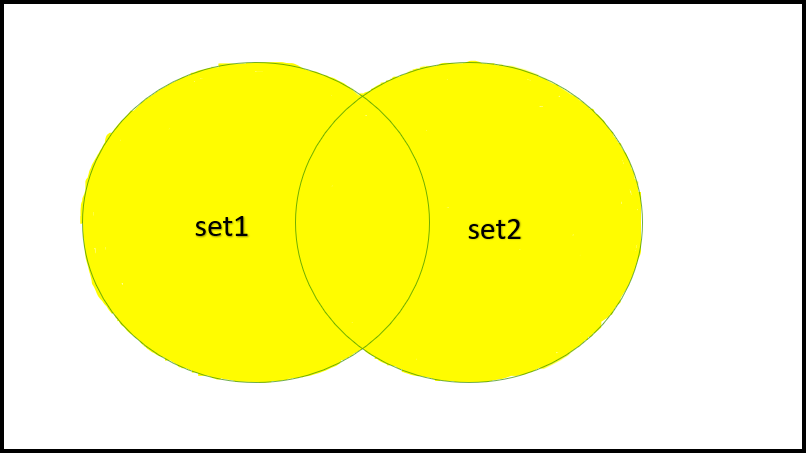Union

Example:

## Swift

 `// Swift program to illustrate the working of Union operation` `// Initializing a set containing multiples of 3``var` `mySet1: Set = [ 3, 6, 9, 12, 15, 18, 21, 24, 27, 30 ]` `// Initializing a set containing multiple of 5``var` `mySet2: Set = [ 5, 10, 15, 20, 25, 30, 35, 40, 45, 50 ]` `// Using inbuilt union method to produce the union``// of sets containing multiples of 3 and 5``var` `unionOfSets = mySet1.union(mySet2).sorted()``print``(``"Union set: \(unionOfSets)"``)`

Output:

`Union set: [3, 5, 6, 9, 10, 12, 15, 18, 20, 21, 24, 25, 27, 30, 35, 40, 45, 50]`

2. Intersection: The intersection of two sets is a set having only the elements common to both sets. For example, consider two sets, mySet1 = {2, 10, 1, 4} and mySet2 = {10, 3, 12}. So the intersection set would be, myIntersection = {10}. In Swift, we can achieve an intersection of two sets with the help of the inbuilt intersection() method used with sets. This method requires two sets and creates a new set containing the intersection of specified sets.

Syntax:

mySet1.intersection(mySet2)

He

mySet1 and mySet2 are initialized sets

Return type: Returns a set having elements that are common to both sets

This operation takes O(n) time, where n is the length of the longest sequence.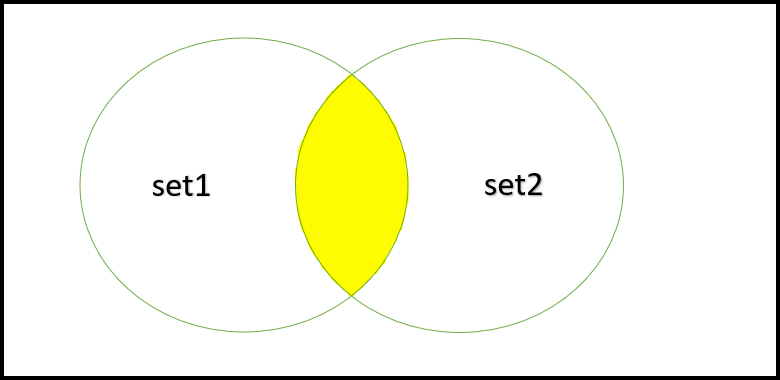Intersection

Example:

## Swift

 `// Swift program to illustrate the working of Intersection set operation` `// Initializing a set containing multiples of 3``var` `mySet1: Set = [ 3, 6, 9, 12, 15, 18, 21, 24, 27, 30 ]` `// Initializing a set containing multiple of 5``var` `mySet2: Set = [ 5, 10, 15, 20, 25, 30, 35, 40, 45, 50 ]` `// Using inbuilt intersection method to produce the``// union of sets containing multiples of 3 and 5``var` `intersectionOfSets = mySet1.intersection(mySet2).sorted()``print``(``"Intersection set: \(intersectionOfSets)"``)`

Output:

`Intersection set: [15, 30]`

3. Subtraction: The subtraction of two sets of the form “set1 – set2” is a set having only the elements which are present in set1 but not present in set2. For example, consider two sets, mySet1 = {2, 10, 1, 4} and mySet2 = {10, 3, 12}. So the subtraction set would be, mySubtraction = {2, 1, 4}. In Swift, we can achieve a subtraction of two sets with the help of the inbuilt subtracting() method used with sets. This method requires two sets and creates a new set containing the subtraction of specified sets.

Syntax:

mySet1.subtracting(mySet2)

Here, mySet1 and mySet2 are initialized sets

Return type: Returns a set having elements that are present in a set (mySet1) but not present in another set (mySet2).

This operation takes O(n) time, wher

is the length of the longest sequence.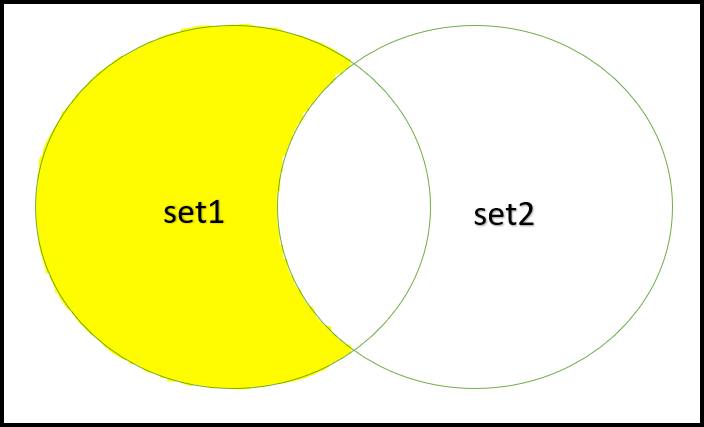Subtraction

Example:

## Swift

 `// Swift program to illustrate the working of``// subtraction set operation` `// Initializing a set containing multiples of 3``var` `mySet1: Set = [ 3, 6, 9, 12, 15, 18, 21, 24, 27, 30 ]` `// Initializing a set containing multiple of 5``var` `mySet2: Set = [ 5, 10, 15, 20, 25, 30, 35, 40, 45, 50 ]` `// Using inbuilt subtracting method to produce the``// subtraction of sets containing multiples of 3 and 5``var` `subtractionOfSets = mySet1.subtracting(mySet2).sorted()``print``(``"Subtraction set: \(subtractionOfSets)"``)`

Output:

`Subtraction set: [3, 6, 9, 12, 18, 21, 24, 27]`

4. Symmetric Difference: The symmetric difference of two sets A and B is a set having all elements of both sets except the common elements. For example, consider two sets, mySet1 = {2, 10, 1, 4} and mySet2 = {10, 3, 12}. So the symmetric difference would be, mySymmetricDifference = {2, 1, 4}. In Swift, we can achieve a symmetric difference of two sets with the help of the inbuilt symmetricDifference() method used with sets. This method requires two sets and creates a new set containing the symmetric difference of specified sets.

Syntax:

mySet1.symmetricDifference(mySet2)

Here, mySet1 and mySet2 are initialized sets

Return type: Returns a set having all elements of both sets but except the common elements.

This operation takes O(n) time, where n is the length of the longest sequence.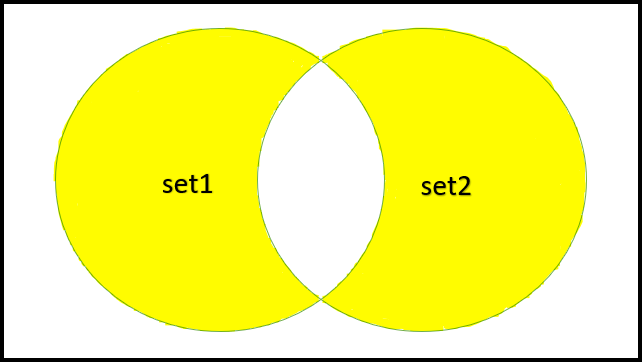Symmetric Difference

Example:

## Swift

 `// Swift program to illustrate the working of``// Symmetric Difference set operation` `// Initializing a set containing multiples of 3``var` `mySet1: Set = [ 3, 6, 9, 12, 15, 18, 21, 24, 27, 30 ]` `// Initializing a set containing multiple of 5``var` `mySet2: Set = [ 5, 10, 15, 20, 25, 30, 35, 40, 45, 50 ]` `// Using inbuilt symmetric difference method to``// produce the symmetric difference of sets``// containing multiples of 3 and 5``var` `symmetricDifferenceOfSets = mySet1.symmetricDifference(mySet2).sorted()``print``(``"Symmetric difference set: \(symmetricDifferenceOfSets)"``)`

Output:

`Symmetric difference set: [3, 5, 6, 9, 10, 12, 18, 20, 21, 24, 25, 27, 35, 40, 45, 50]`

5. Subset: A set is a subset of another set if all elements of the first set are elements of the second set. For example, mySet1 = {1, 2, 4, 3} is a subset of mySet2 = {5, 3 , 1, 2, 4}.  In Swift, we can check whether a set is a subset of another set with the help of the inbuilt isSubset() method used with sets.

Syntax:

mySet1.isSubset(of: mySet2)

Here, mySet1 and mySet2 are initialized sets

Return type:

• true: If a set is a subset of another set
• false: If a set is not a subset of another set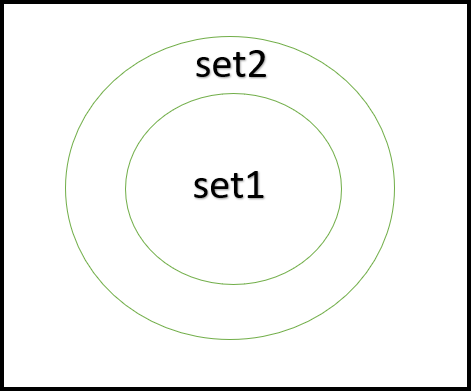Subset

Below is the program to illustrate these set operations:

Example:

## Swift

 `// Swift program to illustrate the working of Subset` `// Initializing a set containing multiples of 3``var` `mySet1: Set = [ 3, 6, 9, 12, 15, 18, 21, 24, 27, 30 ]` `// Initializing a set containing multiple of 5``var` `mySet2: Set = [ 5, 10, 15, 20, 25, 30, 35, 40, 45, 50 ]` `// Check mySet1 is a subset mySet2 or not``print``(``"Is mySet1 a subset of mySet2 ?: \(mySet1.isSubset(of: mySet2))"``)`

Output:

`Is mySet1 a subset of mySet2 ?: false`

### Modifying Sets

To modify a set, Swift provides a number of methods associated with a set and they are:

1. remove(x): This function is used to remove an ‘x‘ element from a Set. If the element is not already present in the Set, even then the program compiles successfully. This is a unique feature in Swift and that is why a set in Swift is sometimes advantageo

ver a set in other languages like C++.

Syntax:

mySet.remove(x)

2. removeFirst(): This method is used to remove the first element of a set. Since set elements are not arranged in any particular order in a set in Swift, that is why we can predict beforehand which element would be removed using this method, The time complexity of this method is Amortized constant, O(1).

Syntax:

mySet.removeFirst(x)

3. removeAll(): This function is used to remove all elements from a set. It takes O(n) time where n is the size of the set.

Syntax:

mySet.removeAll()

4. contains(x): This function is used to check whether the element x is present in the set or not. It takes O(1) time per operation. It will return true if x is present in the set, otherwise, return false if x is not present in the

Syntax:

mySet.contains(x)

5. count: Used to find the number of elements present in a set. It takes O(1) time per operation. For example, we are given a set, mySet = {1, 4, 5, 7, 5}. The count of mySet would be equal to 4. It will always return a non-negative integer.

Syntax:

mySet.count

6. isEmpty: Returns true if a set is empty otherwise returns false. It takes O(1) time per operation. It will return true if mySet is empty, otherwise, return false if mySet is not empty.

Syntax:

mySet.isEmpty

7. sorted(): This function temporarily arranges the elements of the set in sorted order. By temporarily, we mean after this operation the set arranges itself again in random order. It takes O(n log n) time, where n is the length of the set.

Syntax:

e="text-align:justify;">mySet.sorted()

8. firstIndex(x): This method is used to get the hash value of the index of element x. It takes O(1) time per operation.

Syntax:

firstIndex(x)

9. randomElement()!: Returns a random element from the set. It takes O(1) time only if the collection conforms to RandomAccessCollection otherwise it takes O(n) time, where n is the length of the collection.

Syntax:

mySet.randomElement()!

My Personal Notes arrow_drop_up